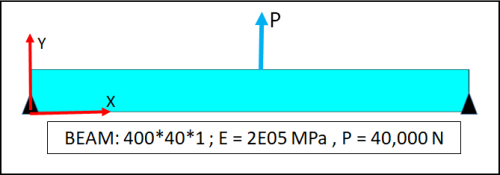## Posts

Showing posts from March, 2021

### Understanding Geometric Nonlinearity - 1 by Kotur RaghavanPreamble Any structure deforms when subjected to loads. The basic question is whether the deformations affect the structural behavior to any practically significant extent. If they do, we know that the small deformation theories are inadequate and it is necessary to invoke large deformation formulation to obtain acceptable results. In other words, one has to invoke the  “Geometric Nonlinearity” option while using FEA packages to obtain good results. The general thumb-rule is that when the deformations are comparable to the dimensions of the structure, the effects of geometric nonlinearity become noticeable. In the small deformation theory or linear elasticity, the basic assumption is that the displacements in orthogonal directions are uncoupled. In Cartesian geometry, the displacement fields X- and Y- directions are independent of one another. In the cylindrical coordinate system, the displacement fields in the radial and tangential directions are assumed to be independent of one